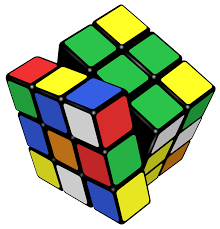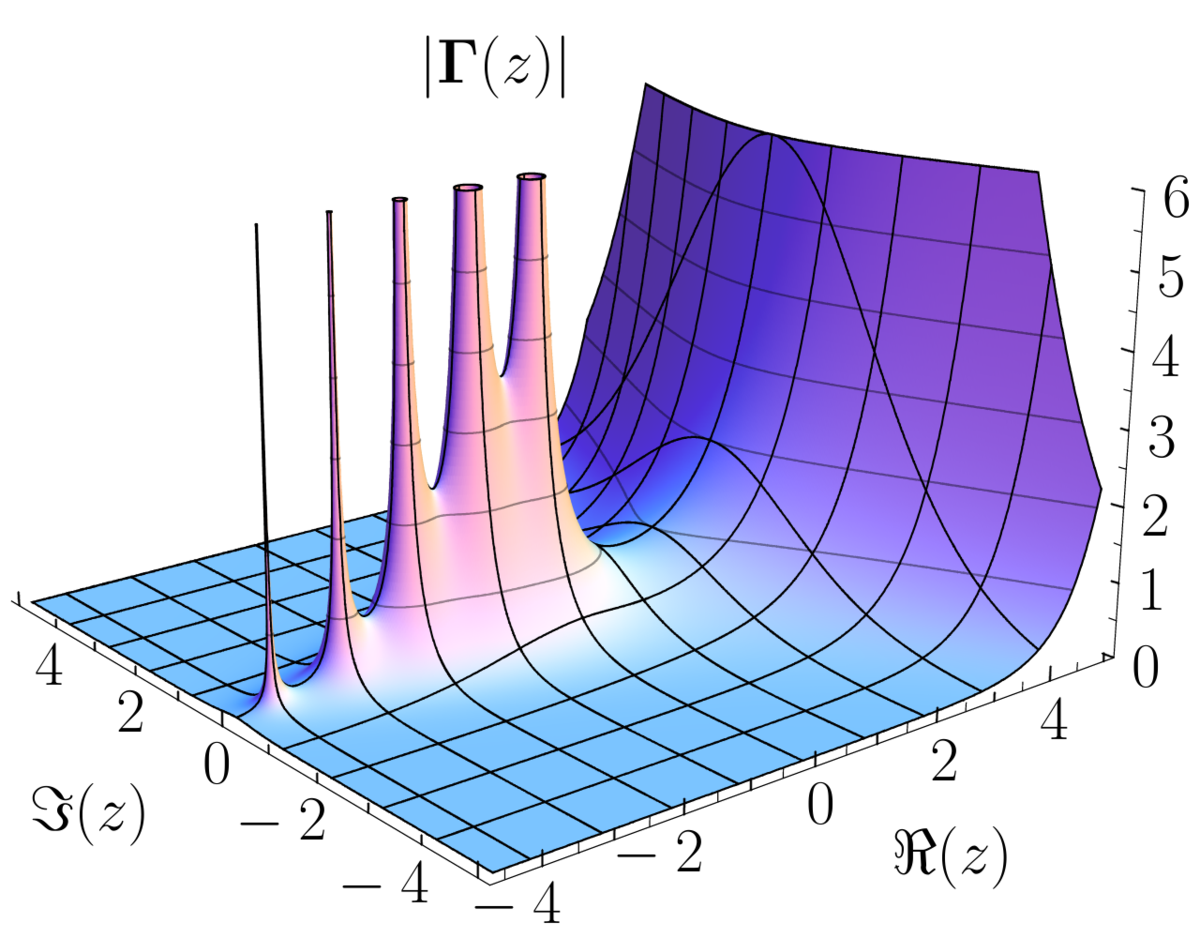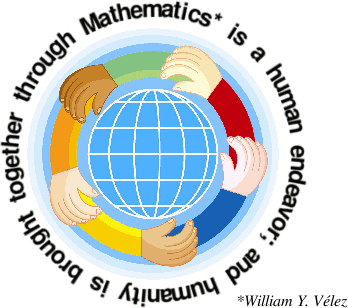#### Number theory-1

This course covers divisibility, congruence,primes and their distribution,Fermat's theorem, Number-theoretic function,primitive roots and indices, Quadratic reciprocity law,Binary quadratic forms, Number of special form,non-linear diophantine equation.#### ALGEBRA-1

abstract algebra (occasionally called modern algebra) is the study of algebraic structures. Algebraic structures include groupsringsfieldsmodulesvector spaceslattices, and algebras.#### Complex Analysis

This course covers

Complex plane, geometric representation of complex numbers, joint equation of circle and straight line, stereographic projection and the spherical representation of the extended complex plane. Topology on the complex plane, connected and simply connected sets. Complex valued functions and their continuity. Curves, connectivity through polygonal lines.Analytic functions, Cauchy-Riemann equations, Harmonic functions and Harmonic conjugates.Power series, exponential and trigonometric functions, arg z, log z, az and their continuous branches.

Complex Integration, line integral, Cauchy’s theorem for a rectangle, Cauchy’s theorem in a disc, index of a point with respect to a closed curve, Cauchy’s integral formula, Higher derivatives, Morrera’s theorem, Liouville’s theorem, fundamental theorem of Algebra. The general form of Cauchy’s theorem.#### Real Analysis - I

This course covers the fundamentals of mathematical analysis : Basic topology, sequences and series, continuity, The Riemann-Stieltjes integral, sequences and series of functions.